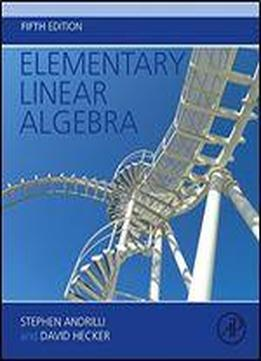Elementary Linear Algebra, Fifth Edition by Stephen Andrilli / 2016 / English / PDF

Elementary Linear Algebra, 5th edition, by Stephen Andrilli and David Hecker, is a textbook for a beginning course in linear algebra for sophomore or junior mathematics majors. This text provides a solid introduction to both the computational and theoretical aspects of linear algebra. The textbook covers many important real-world applications of linear algebra, including graph theory, circuit theory, Markov chains, elementary coding theory, least-squares polynomials and least-squares solutions for inconsistent systems, differential equations, computer graphics and quadratic forms. Also, many computational techniques in linear algebra are presented, including iterative methods for solving linear systems, LDU Decomposition, the Power Method for finding eigenvalues, QR Decomposition, and Singular Value Decomposition and its usefulness in digital imaging. The most unique feature of the text is that students are nurtured in the art of creating mathematical proofs using linear algebra as the underlying context. The text contains a large number of worked out examples, as well as more than 970 exercises (with over 2600 total questions) to give students practice in both the computational aspects of the course and in developing their proof-writing abilities. Every section of the text ends with a series of true/false questions carefully designed to test the students’ understanding of the material. In addition, each of the first seven chapters concludes with a thorough set of review exercises and additional true/false questions. Supplements to the text include an Instructor’s Manual with answers to all of the exercises in the text, and a Student Solutions Manual with detailed answers to the starred exercises in the text. Finally, there are seven additional web sections available on the book’s website to instructors who adopt the text. Builds a foundation for math majors in reading and writing elementary mathematical proofs as part of their intellectual/professional development to assist in later math coursesPresents each chapter as a self-contained and thoroughly explained modular unit.Provides clearly written and concisely explained ancillary materials, including four appendices expanding on the core concepts of elementary linear algebraPrepares students for future math courses by focusing on the conceptual and practical basics of proofs

views: 1529